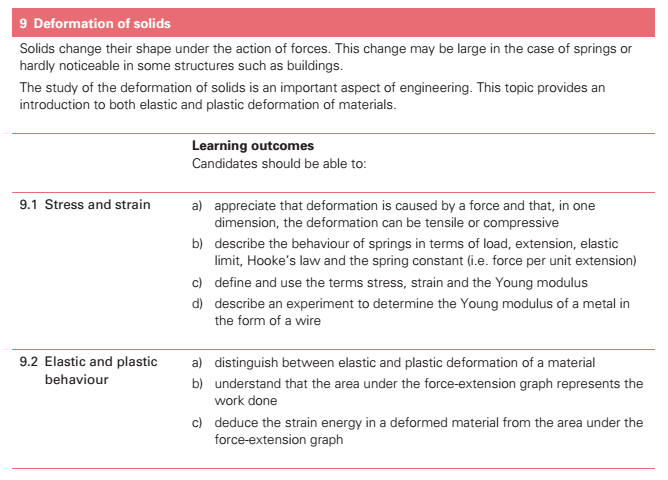9. Deformation of solids (a) appreciate that deformation is caused by a force and that, in one dimension, the deformation can be tensile or compressive. (b) describe the behaviour of springs in terms of load, extension, elastic limit, Hooke’s law and the spring constant[] (i.e. force per unit extension). (c) define and use the terms stress, strain and the Young modulus. (d) describe an experiment to determine the Young modulus of a metal in the form of a wire. (e) distinguish between elastic and plastic deformation of a material. (f) deduce the strain energy in a deformed material from the area under the force-extension graph. (g) demonstrate knowledge of the force-extension graphs for typical ductile, brittle and polymeric materials, including an understanding of ultimate tensile stress. Alpha-particle scattering is studied as evidence for the structure of the atom. Nuclear composition, in terms of nucleons, leads to an appreciation of mass defect and binding energy[].  Nuclear processes including radioactive decay, fission and fusion are studied. An introduction to fundamental particles is included.